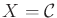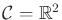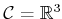# 13.1 Velocity Constraints on the Configuration Space

In this section, it will be assumed that, which is a C-space of one or more rigid bodies, as defined in Section 4.2. Differential models in this section are all expressed as constraints on the set of allowable velocities at each point in. This results in first-order differential equations because only velocities are constrained, as opposed to accelerations or higher order derivatives.

To carefully discuss velocities, it will be assumed thatis a smooth manifold, as defined in Section 8.3.2, in addition to a topological manifold, as defined in Section 4.1.2. It may be helpful to keep the casesandin mind. The velocities are straightforward to define without resorting to manifold technicalities, and the dimension is low enough that the concepts can be visualized.

Subsections

Steven M LaValle 2020-08-14